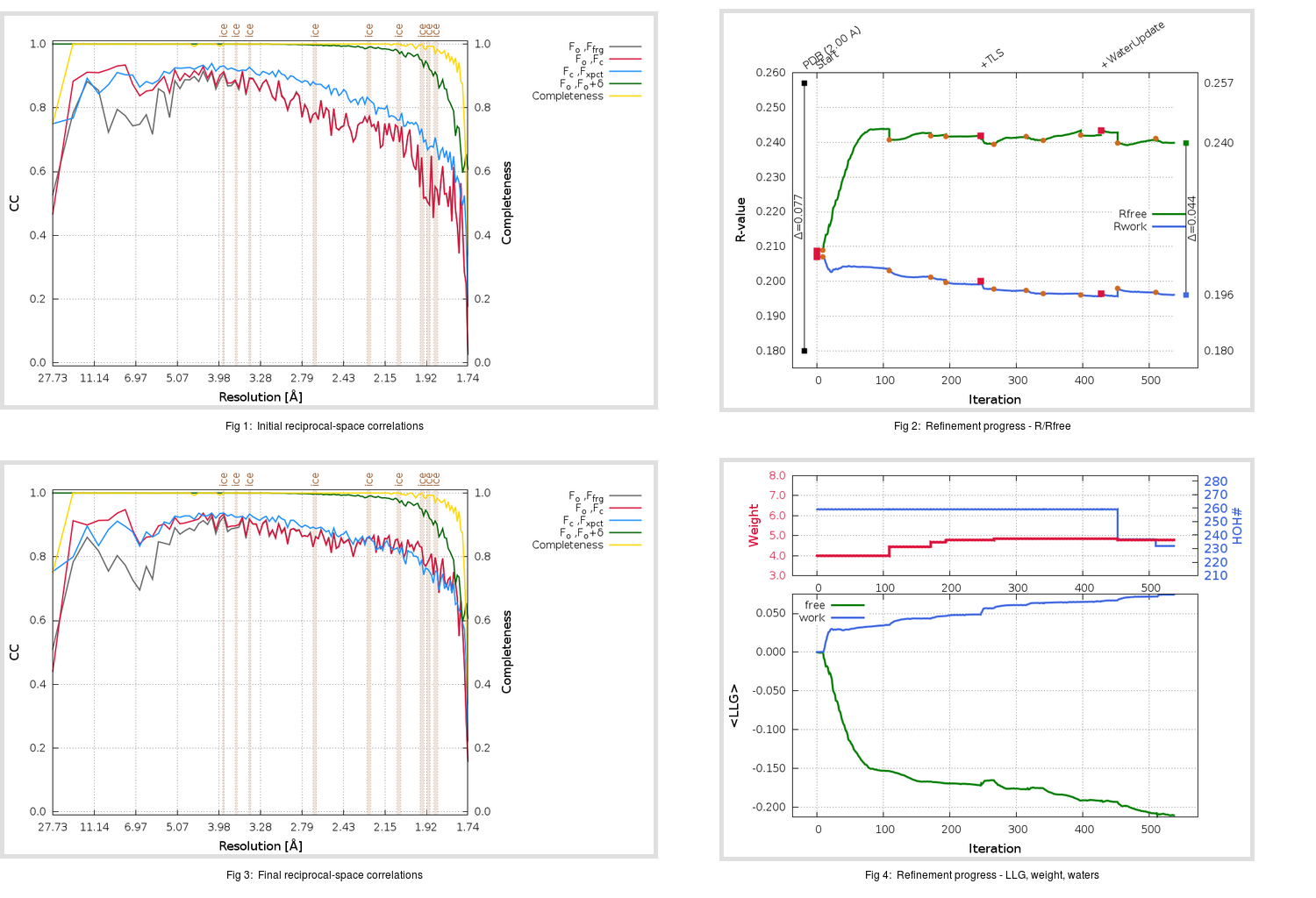Content:

```    Diffraction limits & principal axes of ellipsoid fitted to diffraction cut-off surface:
1.735         1.0000   0.0000   0.0000       a*
1.735         0.0000   1.0000   0.0000       b*
1.738         0.0000   0.0000   1.0000       c*
```

## Deposited

` `
 Date deposited Date data collection Resolution R, Rfree 20050722 20041111 2.00 0.1750 0.2570

Molprobity (CCP4 7.0 version) summary:

```Ramachandran outliers =   1.34 %
favored =  95.97 %
Rotamer outliers      =   3.70 %
C-beta deviations     =     2
Clashscore            =  11.90
RMS(bonds)            =   0.0181
RMS(angles)           =   1.71
MolProbity score      =   2.30
Resolution            =   2.00
R-work                =   0.1750
R-free                =   0.2570
```

```Number of waters      =   259

<B> (all atoms) =   23.77 ( sd =    8.18 ) for       2672 non-hydrogen atoms
<B>   (protein) =   22.63 ( sd =    7.08 ) for       2413 non-hydrogen atoms
<B>     (water) =   34.17 ( sd =    9.62 ) for        259 non-hydrogen atoms
<B>    (others) =    0.00 ( sd =    0.00 ) for          0 non-hydrogen atoms

B min/max       (all non-hydrogen atoms) =   10.08 /   59.42
B min/max   (protein non-hydrogen atoms) =   10.08 /   55.97
B min/max     (water non-hydrogen atoms) =   11.91 /   59.42
B min/max     (other non-hydrogen atoms) =    0.00 /    0.00
```

## BUSTER (re-)refinement

` `

Molprobity (CCP4 7.0 version) summary:

```Ramachandran outliers =   0.67 %
favored =  96.31 %
Rotamer outliers      =   2.22 %
C-beta deviations     =     0
Clashscore            =   2.71
RMS(bonds)            =   0.0114
RMS(angles)           =   1.59
MolProbity score      =   1.57
Resolution            =   1.74
R-work                =   0.1961
R-free                =   0.2398
```

```Number of waters      =   232

<B> (all atoms) =   26.23 ( sd =    9.35 ) for       2645 non-hydrogen atoms
<B>   (protein) =   25.10 ( sd =    8.31 ) for       2413 non-hydrogen atoms
<B>     (water) =   37.68 ( sd =   10.98 ) for        232 non-hydrogen atoms
<B>    (others) =    0.00 ( sd =    0.00 ) for          0 non-hydrogen atoms

B min/max       (all non-hydrogen atoms) =   10.59 /   78.20
B min/max   (protein non-hydrogen atoms) =   10.59 /   54.34
B min/max     (water non-hydrogen atoms) =   14.91 /   78.20
B min/max     (other non-hydrogen atoms) =    0.00 /    0.00
```

Refinement progression:Results:

` `
 File Remark 2BX3_aB_refine.01_03_refine.pdb.gz exact refinement commands are in header 2BX3_aB_refine.01_03_refine.mtz.gz including original deposited data and several re-refinement map coefficients 2BX3_aB_refine.01_03_BUSTER_model.cif.gz including any non-standard compound restraints 2BX3_aB_refine.01_03_BUSTER_refln.cif.gz